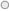### Numeracy LabIntegers are whole numbers which can be represented as positive, negative and zero.

Integers are interesting number system that is applicable in banking to show the debt of loan remaining, temperature readings on the thermometer and altitude below sea level.

In working with integers word problems we have to know these terms that represent positive and negative integers.

Terms used as positive integers:

 ·         Earned Increase ·         Save Up ·         Deposit Ascend ·         Gained Profit ·         Won Climbed ·         Rose

Terms used as negative integers:

 ·         Spent Cost ·         Withdraw Lost ·         Fell Decrease ·         Down Descent ·         Loss Dove

Life examples of integers in banking:http://news.pngfacts.com/2017/02/bank-south-pacific-to-introduce-deposit.html

Jane has deposited K100 to his BSP account using the deposit ATM.

Life example of integers in altitude:https://en.wikipedia.org/wiki/Mount_Wilhelm

The highest mountain in Papa New Guinea is Mt- Wilhelm of 4,509 meters above sea level.

In arithmetic operation involving integers, they are some rules that we have to bear in mind while performing those operations.

Rule 1: Same Signs – Add and keep the sign

·         Add the integer and keep the sign.

(positive Z) + (positive Z) = Positive Z

For example:

a. (-4) + (+5) = 9

b)     (-4) + (-5) = -9

(negative Z) + (negative Z) = Negative Z

Rule 2: Different Signs – Subtract and keep the sign of the bigger number

·         Subtract the absolute value of the numbers and keep the sign of the bigger number.

For example:

a)     (-4) + (+5) = 1

b)     (+4) + (-5) = -1

Subtraction of integer:

Rule: Do not subtract the integers. You must change the signs.

·         Keep the sign of the first number

·         Change the subtraction sign to addition

·         Change the sign of the second number to the opposite sign. If it is positive, change to negative. If it is negative change to positive.

For example:

a)     (+4) – (-4)

(+4) + (4) = 8

b)     (-8) – (4)

(-8) + (-4) = -12

Multiplication of integers:

Rule 1: Same Sign = Positive

Multiply the number. Answers will be positive.

For example:

(-5) x (-5) = 25

Rule 2: Different Sign = Negative

Multiply the numbers. Answers will be negative

For example:

(+5) x ( -5) = -25

Division of integers:

Rule 1: Same Sign = Positive

Divide the number. Answers will be positive.

For example:

(-5)   (-5) = 25

Rule 2: Different Sign = Negative

Divide the number. Answers will be negative

For example:

(5)   (-5) = -25